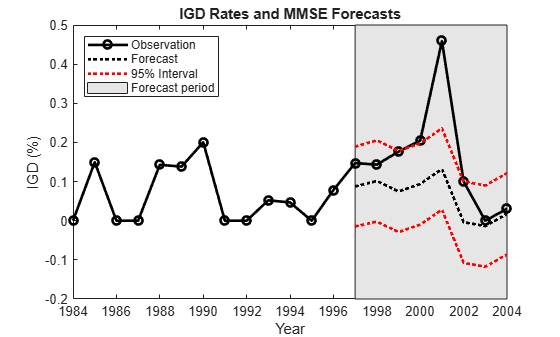# Forecast IGD Rate from ARX Model

This example shows how to generate minimum mean square error (MMSE) and Monte Carlo forecasts from an ARX(1) model fitted to historical data. The response series contains annual measurements of the default rate on investment-grade corporate bonds (`IGD`).

A dynamic model requires initial values for estimation and forecasting. Also, to include exogenous effects in the forecasts, a model containing a regression component requires exogenous data in the forecast period. Therefore, this example shows how to partition the sample timeline into presample, estimation, and forecast periods to obtain the required data.

Load the data set containing the credit default rates.

`load Data_CreditDefaults`

For details on the data set, display `Description`.

The table `DataTable` contains yearly measurements, but the data set is agnostic of the time base. To apply the time base to the data, convert `DataTable` to a timetable.

`Tbl = table2timetable(DataTable,"RowTimes",datetime(DataTable.Dates,"Format","yyyy"));`

Econometrics Toolbox™ `arima` software removes missing observations (indicated by `NaN`s) from the data by using list-wise deletion. This action affects how you partition data.

Determine whether any row in the data contains at least one missing observation.

```idxmiss = any(ismissing(Tbl),2); anymissing = sum(idxmiss)```
```anymissing = 0 ```

There are no missing observations in the data.

Determine the total sample size.

`T = size(Tbl,1)`
```T = 21 ```

### Partition Timeline

Assume all series in the data are stationary, and consider this ARX(1) model for the IGD rate:

`${\text{IGD}}_{t}=c+{\text{IGD}}_{t-1}+{\beta }_{1}{\text{AGE}}_{t}+{\beta }_{2}{\text{CPF}}_{t}+{\beta }_{3}{\text{SPR}}_{t}+{\epsilon }_{t},$`

where ${\epsilon }_{\mathit{t}}$ is an iid series of Gaussian random variables with a mean of 0 and a finite variance.

To estimate an ARX(1) model, the conditional mean of the first response requires the previous response. Therefore, the presample period for estimation is the first row of the data. Also, consider forecasting the IGD into an 8-year forecast horizon and comparing the forecasts to the observed responses. Therefore, the forecast period is the last eight rows of the data. Fit the model to the remaining data.

Create index vectors that identify the presample, estimation, and forecast periods.

```fh = 8; % Forecast horizon idxpre = 1; idxest = 2:(T - fh); idxf = (T - fh + 1):T; fprintf('\nPresample period: %d\nEstimation Period: %d - %d\nForecast period: %d - %d\n',... year(Tbl.Time([idxpre idxest(1) idxest(end) idxf(1) idxf(end)])))```
```Presample period: 1984 Estimation Period: 1985 - 1996 Forecast period: 1997 - 2004 ```

### Fit Model to Data

Create an `arima` model object that represents an ARX(1) model.

`Mdl = arima(1,0,0)`
```Mdl = arima with properties: Description: "ARIMA(1,0,0) Model (Gaussian Distribution)" Distribution: Name = "Gaussian" P: 1 D: 0 Q: 0 Constant: NaN AR: {NaN} at lag  SAR: {} MA: {} SMA: {} Seasonality: 0 Beta: [1×0] Variance: NaN ```

`Mdl` is a template for estimation. Properties of `Mdl` that have the value `NaN` correspond to unknown ARX(1) model parameters to be estimated by `estimate`. Although the regression coefficient property `Beta` is empty, `estimate` determines its size by the number of columns in the specified exogenous data matrix.

Fit the model to the estimation sample response and exogenous data. Specify the presample response.

```X = Tbl{:,["AGE" "CPF" "SPR"]}; Mdl = estimate(Mdl,Tbl.IGD(idxest),'Y0',Tbl.IGD(idxpre),... 'X',X(idxest,:));```
``` ARIMAX(1,0,0) Model (Gaussian Distribution): Value StandardError TStatistic PValue __________ _____________ __________ ________ Constant 0.022517 0.20278 0.11104 0.91158 AR{1} -0.18486 0.43861 -0.42146 0.67342 Beta(1) 0.0091356 0.016249 0.56224 0.57395 Beta(2) -0.0098351 0.0053577 -1.8357 0.066402 Beta(3) 0.0039044 0.057031 0.068461 0.94542 Variance 0.0027082 0.0014648 1.8489 0.064468 ```

`Mdl` is a fully specified `arima` model object representing a fitted ARX(1) model. The estimation summary suggests that all variables except the `CPF` (`Beta(2)`) are insignificant at a 10% level of significance.

### Generate MMSE Forecasts

Forecast MMSE responses into an 8-year forecast horizon and compute corresponding forecast error variances. Because the 1-period-ahead forecast ${\underset{}{\overset{ˆ}{y}}}_{1997}$ requires the presample response ${\mathit{y}}_{1996}$ and the $\mathit{t}$-period-ahead forecast requires exogenous data ${\mathit{X}}_{\mathit{t}}$, specify the final response in the estimation sample as a presample response, and specify the forecast period exogenous data.

```[yf,ymse] = forecast(Mdl,fh,Tbl.IGD(idxest(end)),... 'XF',X(idxf,:));```

Calculate prediction intervals for the forecasts assuming that they are normally distributed.

`ci = yf + 1.96*[-sqrt(ymse) sqrt(ymse)];`

### Plot MMSE Forecasts

Plot the response series using their MMSE forecasts and prediction intervals.

```figure h1 = plot(Tbl.Time,Tbl.IGD,'ko-','LineWidth',2); hold on h2 = plot(Tbl.Time(idxf),yf,'k:','LineWidth',2); h3 = plot(Tbl.Time(idxf),ci,'r:','LineWidth',2); h = gca; px = Tbl.Time(idxf([1 end end 1])); py = h.YLim([1 1 2 2]); hf = fill(px,py,[0.9 0.9 0.9]); uistack(hf,"bottom"); legend([h1 h2 h3(1) hf],["Observation" "Forecast"... "95% Interval" "Forecast period"],'Location','NorthWest') title('IGD Rates and MMSE Forecasts') xlabel('Year') ylabel('IGD (%)') axis tight hold off```The forecasts seem reasonable despite the extreme observation occurring in 2001.

### Generate Monte Carlo Forecasts

Forecast responses by simulating 100 paths from the fitted ARX(1) model. Then, aggregate the random paths by computing the mean and the 2.5% and 97.5% percentile intervals at each time point in the forecast period. Similar to MMSE forecast estimation, `simulate` requires the presample response ${\mathit{y}}_{1996}$ to generate ${\mathit{y}}_{1997}$ from the fitted ARX(1) model, and it requires exogenous data in the forecast period.

```nsim = 100; rng(1); YMC = simulate(Mdl,fh,'NumPaths',nsim,... 'Y0',Tbl.IGD(idxest(end)),'X',X(idxf,:)); YMCbar = mean(YMC,2); YMCci = quantile(YMC',[0.025 0.975]);```

### Plot Monte Carlo Forecasts

Plot the response series with the Monte Carlo forecasts and 95% percentile intervals.

```figure h1 = plot(Tbl.Time,Tbl.IGD,'ko-','LineWidth',2); hold on h2 = plot(Tbl.Time(idxf),YMC,'Color',[0.7 0.7 0.7]); h3 = plot(Tbl.Time(idxf),YMCbar,'c:','LineWidth',3); h4 = plot(Tbl.Time(idxf),YMCci,'r:','LineWidth',3); h = gca; px = Tbl.Time(idxf([1 end end 1])); py = h.YLim([1 1 2 2]); hf = fill(px,py,[0.9 0.9 0.9]); uistack(hf,"bottom"); legend([h1 h2(1) h3 h4(1) hf],["Observation" "Monte Carlo path"... "Monte Carlo mean" "95% Interval" "Forecast period"],'Location','NorthWest') title('IGD Rates and Monte Carlo Forecasts') xlabel('Year') ylabel('IGD (%)') axis tight hold off```The Monte Carlo forecasts and prediction intervals resemble those from the MMSE forecasting.

 Helwege, J., and P. Kleiman. "Understanding Aggregate Default Rates of High Yield Bonds." Current Issues in Economics and Finance. Vol. 2, No. 6, 1996, pp. 1-6.

 Loeffler, G., and P. N. Posch. Credit Risk Modeling Using Excel and VBA. West Sussex, England: Wiley Finance, 2007.